RadPdfProcessing allows you to create pdf files that contain text, images and any kind of custom shapes. You can export this document, protect it with a password and import it back.

This example will show you how to create a document which displays information about some controls and their usage. (Theoretically we could have gotten this information using Telerik Analytcs.) Here is how our final report will look like: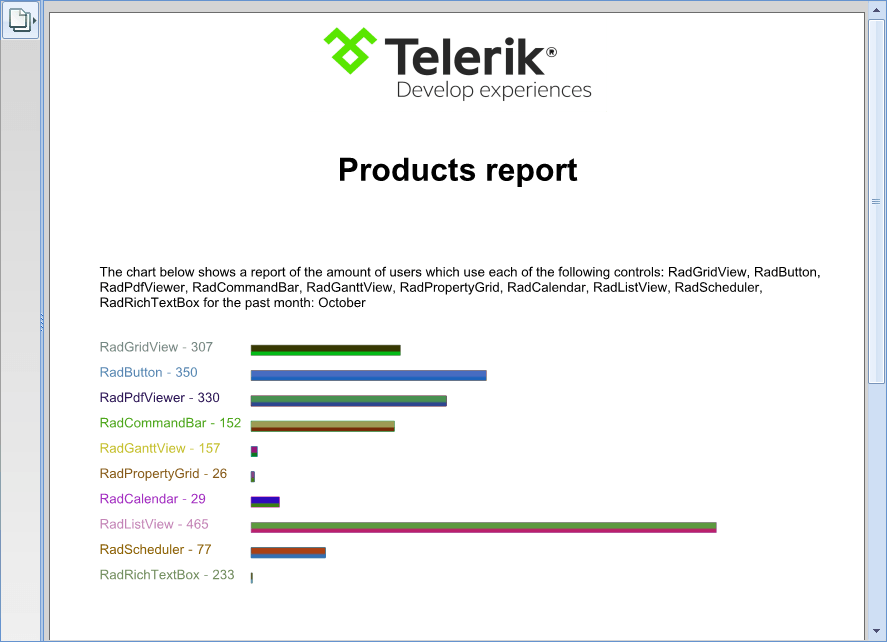First thing's first. We need to create a WinForms project and add the assemblies of the RadPdfProcessing library, listed below (available in our Getting Started help article as well):

• Telerik.Windows.Documents.Core.dll
• Telerik.Windows.Documents.Fixed.dll
• Telerik.Windows.Zip.dll

You will also need to add a reference to WindowsBase, because the library uses some of the types in it.

Hint: The Telerik assemblies can be found in the installation of the UI for WinForms suite or in GAC and the WindowsBase is in the Assemblies -> Framework tab in the Reference Manager (the window which pops up after ‘Add reference’ is clicked)

If at this point, your project is building without errors. This means you have the needed references in place and we can proceed with writing some actual code. Since we will need data to work with, we can create a quick ReportGenerator, which will generate some random data for us. The model we are going to use will be called ControlUsage:

`public` `class` `ControlUsage`
`{`
`    ``public` `string` `Name { ``get``; ``set``; }`
`    ``public` `int` `AmountOfUsers { ``get``; ``set``; }`
`}`
`public` `static` `class` `ReportGenerator`
`{`
`    ``private` `static` `Random random = ``new` `Random();`
`    ``private` `static` `string``[] availableControls = ``new` `string``[] {`
`        ``"RadGridView"``,`
`        ``"RadButton"``,`
`        ``"RadPdfViewer"``,`
`        ``"RadCommandBar"``,`
`        ``"RadGanttView"``,`
`        ``"RadPropertyGrid"``,`
`        ``"RadCalendar"``,`
`        ``"RadListView"``,`
`        ``"RadScheduler"``,`
`        ``"RadRichTextBox"`
`    ``};`

`    ``public` `static` `List<ControlUsage> Generate()`
`    ``{`
`        ``List<ControlUsage> report = ``new` `List<ControlUsage>();`

`        ``for` `(``int` `i = 0; i < availableControls.Length; i++)`
`        ``{`
`            ``report.Add(``new` `ControlUsage`
`                ``{`
`                    ``AmountOfUsers = random.Next(20, 500),`
`                    ``Name = availableControls[i]`
`                ``});`
`        ``}`

`        ``return` `report;`
`    ``}`
`}`

Nothing fancy here; just associating a random number with a string and putting it all into an object. Let’s head on to our form and generate some reports:

`public` `Form1()`
`{`
`    ``InitializeComponent();`

`    ``List<ControlUsage> report = ReportGenerator.Generate();`
`}`

Having our data, we need to put it in use. What better way than creating a class called
ReportWriter. For now, we will declare some basic fields, properties and methods:

`public` `class` `ReportWriter`
`    ``{`
`        ``private` `RadFixedDocument document;`
`        ``private` `FixedContentEditor editor;`
`        ``private` `Random random;`

`        ``public` `IList<ControlUsage> Report { ``get``; ``private` `set``; }`
`        ``public` `int` `VerticalItemsOffset { ``get``; ``set``; }`
`        ``public` `int` `HorizontalItemsOffset { ``get``; ``set``; }`
`        ``public` `int` `LeftPadding { ``get``; ``set``; }`

`        ``public` `ReportWriter(IList<ControlUsage> report)`
`        ``{`
`            ``this``.Report = report;`
`            ``this``.VerticalItemsOffset = 10;`
`            ``this``.HorizontalItemsOffset = 10;`
`            ``this``.LeftPadding = 50;`
`            ``this``.random = ``new` `Random();`
`        ``}`

`        ``public` `RadFixedDocument WriteToDocument()`
`        ``{`
`            ``this``.document = ``new` `RadFixedDocument();`
`            ``RadFixedPage page = ``this``.document.Pages.AddPage();`
`            ``this``.editor = ``new` `FixedContentEditor(page);`

`            ``this``.DrawLogo();`
`            ``this``.DrawHeading();`
`            ``this``.DrawText();`
`            ``this``.DrawChart();`

`            ``return` `this``.document;`
`        ``}`
`}`

What you can see is that it has a document and an editor. They will be used, along with the random generator (to create some pretty colors) in the process. The offsets will be used for spacing between items, and the WriteToDocument method will actually create the document by calling some private methods. You can also see that it is adding a page to the newly created document. This is the page we will be drawing on.

Maybe you noticed the missing methods: DrawLogo, DrawHeading, DrawText and DrawChart.

Okay, first the logo. For this we can use the Image help article in order to get the idea behind the images. Overall, we simply need to load our image into a stream, create an Telerik.Windows.Documents.Fixed.Model.Objects.Image object and add it to the page:

`private` `void` `DrawLogo()`
`{`
`    ``System.Reflection.Assembly myAssembly = System.Reflection.Assembly.GetExecutingAssembly();`
`    ``Stream myStream = myAssembly.GetManifestResourceStream(``"DplPdfProcessing.logo.png"``);`

`    ``Telerik.Windows.Documents.Fixed.Model.Objects.Image image = ``new` `Telerik.Windows.Documents.Fixed.Model.Objects.Image();`
`    ``image.ImageSource = ``new` `Telerik.Windows.Documents.Fixed.Model.Resources.ImageSource(myStream);`
`    ``image.Width = 300;`
`    ``image.Height = 100;`
`    ``image.Position.Translate(``this``.document.Pages.First().Size.Width / 2 - image.Width / 2, 0);`

`    ``this``.document.Pages.First().Content.Add(image);`
`}`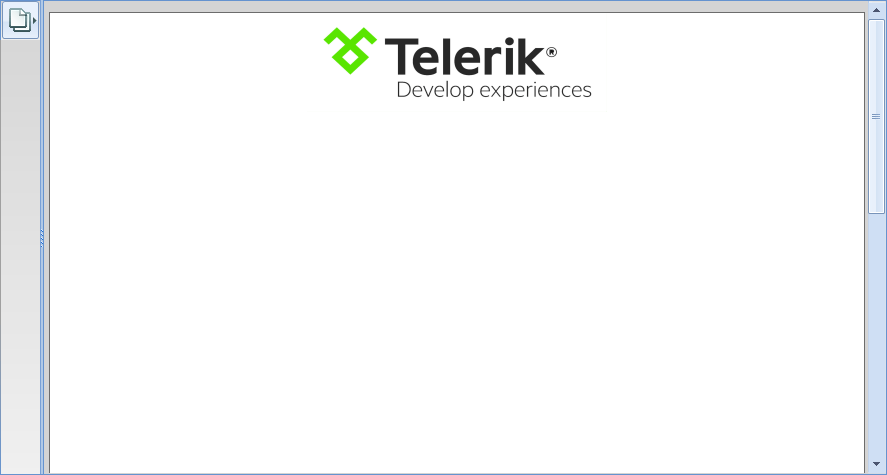Okay, that was easy. Let’s head to the next method–DrawHeading. Here, we are going to use a FixedContentEditor, which allows us to easily set different font, font size and alignment prior to drawing text. Also, before drawing anything with the editor, we must ensure that it is on the correct position. That is why we need to use the Translate method on its Position:

`private` `void` `DrawHeading()`
`{`
`    ``using` `(``this``.editor.SaveProperties())`
`    ``{`
`        ``Block textBlock = ``new` `Block();`
`        ``textBlock.TextProperties.Font = FontsRepository.ZapfDingbats;`
`        ``textBlock.TextProperties.FontSize = 40;`
`        ``textBlock.HorizontalAlignment = Telerik.Windows.Documents.Fixed.Model.Editing.Flow.HorizontalAlignment.Center;`

`        ``string` `text = ``"Products report"``;`
`        ``textBlock.InsertText(text);`
`        ``double` `x = (``this``.document.Pages.First().Size.Width / 2) - (textBlock.Measure().Width / 2);`
`        ``this``.editor.Position.Translate(x, textBlock.Measure().Height + 100);`

`        ``editor.DrawBlock(textBlock);`
`    ``}`
`}`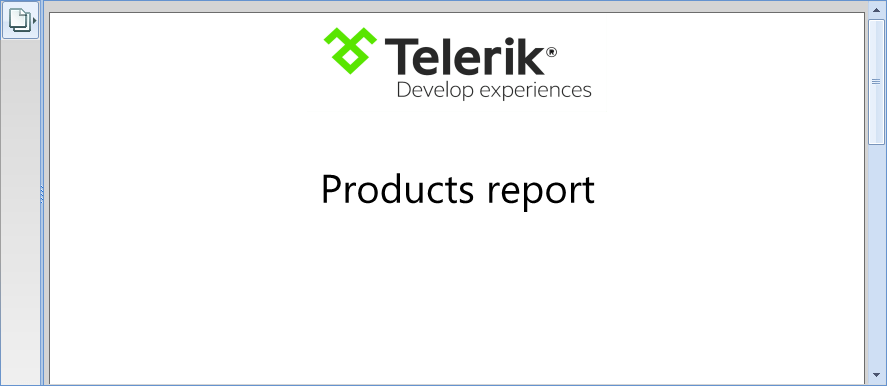Afterward, we need to let the readers of the document know what they are actually looking at. So, let's add some text that clarifies the situation a bit. This is where our DrawText method comes into play:

`private` `void` `DrawText()`
`{`
`    ``using` `(``this``.editor.SaveProperties())`
`    ``{`
`        ``this``.editor.Position.Translate(``this``.LeftPadding, 250);`
`        ``this``.editor.TextProperties.Font = FontsRepository.HelveticaOblique;`
`        ``Block block = ``new` `Block();`

`        ``this``.editor.PushClipping(``new` `Rect(``this``.document.Pages.First().Size));`

`        ``string` `allControls = ``string``.Join(``", "``, ``this``.Report.Select(x => x.Name).ToArray());`
`        ``string` `lastMonth = DateTime.Today.AddMonths(-1).ToString(``"MMMM"``, CultureInfo.InvariantCulture);`
`        ``string` `text = ``string``.Format(``"The chart below shows a report of the amount of users which use each of the following controls: {0} for the past month: {1}"``, allControls, lastMonth);`
`        ``block.InsertText(text);`

`        ``this``.editor.DrawBlock(block, ``new` `Size(``this``.document.Pages.First().Size.Width - ``this``.LeftPadding, ``this``.document.Pages.First().Size.Height));`
`        ``this``.editor.PopClipping(); ``//clipping is not preserved`
`    ``}`
`}`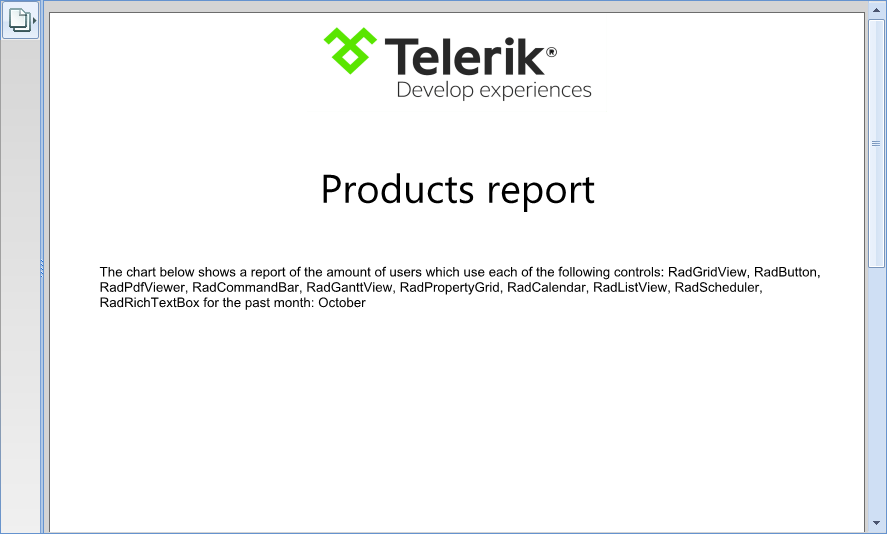Finally, before our report is ready, the actual chart must be created. Since this is the biggest part, I will split it. First, we need to know how big our chart will be. Here is how we will get its dimensions:

`private` `Rect GetChartDimensions(Point start)`
`{`
`    ``Block textBlock = ``new` `Block();`
`    ``textBlock.InsertText(``this``.Report.First().Name);`
`    ``double` `textHeight = textBlock.Measure().Height;`
`    ``double` `chartHeight = (textHeight + ``this``.VerticalItemsOffset) * ``this``.Report.Count;`

`    ``double` `pageWidth = ``this``.document.Pages.First().Size.Width;`
`    ``double` `chartWidth = pageWidth * 0.55;`

`    ``Point leftBottomPoint = ``new` `Point(start.X, start.Y + chartHeight);`
`    ``Point rightTopPoint = ``new` `Point(chartWidth + start.X, start.Y);`
`    ``Point rightBottomPoint = ``new` `Point(chartWidth + start.X, chartHeight + start.Y);`

`    ``Rect chartRect = ``new` `Rect(start, ``new` `Size(rightTopPoint.X - start.X, chartHeight));`

`    ``return` `chartRect;`
`}`

Since the colors of the bars and the text will be different, we can use our
Random object to generate them:

`private` `RgbColor RandomRgbColor()`
`{`
`    ``return` `new` `RgbColor(``this``.RandomRgbValue(), ``this``.RandomRgbValue(), ``this``.RandomRgbValue());`
`}`

`private` `byte` `RandomRgbValue()`
`{`
`    ``return` `(``byte``)``this``.random.Next(0, 201);`
`}`

Now, we have our random color generator and we know how big our chart has to be. What’s left is to actually create it:

`private` `void` `DrawChart()`
`{`
`    ``this``.editor.TextProperties.Font = FontsRepository.Courier;`
`    ``double` `maxWidth = ``this``.Report.Max(x =>`
`        ``{`
`            ``Block block = ``new` `Block();`
`            ``block.InsertText(x.Name);`
`            ``return` `block.Measure().Height;`
`        ``});`

`    ``Point chartStartPoint = ``new` `Point(maxWidth, 250);`

`    ``Rect chartDimensions = ``this``.GetChartDimensions(chartStartPoint);`

`    ``this``.editor.Position.Translate(``this``.LeftPadding, 325);`
`    ``int` `maxValue = ``this``.Report.Max(x => x.AmountOfUsers);`
`    ``Block textBlock = ``new` `Block();`
`    ``textBlock.InsertText(``this``.Report.First().Name);`
`    ``double` `textHeight = textBlock.Measure().Height;`
`    ``double` `startX = 0;`

`    ``for` `(``int` `i = 0; i < ``this``.Report.Count; i++)`
`    ``{`
`        ``ControlUsage item = ``this``.Report[i];`
`        ``Block measureBlock = ``new` `Block();`
`        ``measureBlock.InsertText(``string``.Format(``"{0} - {1}"``, item.Name, item.AmountOfUsers));`
`        ``Size measuredSize = measureBlock.Measure();`
`        ``startX = Math.Max(startX, measuredSize.Width);`
`    ``}`

`    ``for` `(``int` `i = 0; i < ``this``.Report.Count; i++)`
`    ``{`
`        ``this``.DrawBarAndText(maxValue, textHeight, startX, ``this``.Report[i]);`
`    ``}`
`}`

`private` `void` `DrawBarAndText(``int` `maxValue, ``double` `textHeight, ``double` `startX, ControlUsage item)`
`{`
`    ``string` `name = item.Name;`

`    ``RgbColor fillColor = ``this``.RandomRgbColor();`
`    ``Block textBlock = ``new` `Block();`
`    ``this``.editor.GraphicProperties.FillColor = fillColor;`
`    ``this``.editor.GraphicProperties.StrokeColor = ``new` `RgbColor(fillColor.B, fillColor.G, fillColor.R);`
`    ``textBlock.GraphicProperties.CopyFrom(``this``.editor.GraphicProperties);`
`    ``textBlock.InsertText(``string``.Format(``"{0} - {1}"``, name, item.AmountOfUsers));`
`    ``this``.editor.DrawBlock(textBlock);`

`    ``Size measuredText = textBlock.Measure();`
`    ``int` `width = item.AmountOfUsers - (maxValue % item.AmountOfUsers);`
`    ``this``.editor.Position.Translate(``this``.editor.Position.Matrix.OffsetX + startX, ``this``.editor.Position.Matrix.OffsetY);`

`    ``LinearGradient linearGradient = ``new` `Telerik.Windows.Documents.Fixed.Model.ColorSpaces.LinearGradient(``new` `Point(0, 0), ``new` `Point(30, 30));`
`    ``linearGradient.GradientStops.Add(``new` `GradientStop(``this``.RandomRgbColor(), 0));`
`    ``linearGradient.GradientStops.Add(``new` `GradientStop(``this``.RandomRgbColor(), 1));`

`    ``this``.editor.GraphicProperties.FillColor = linearGradient;`
`    ``IPosition savedPosition = ``this``.editor.Position.Clone();`
`    ``this``.editor.Position.Translate(0, ``this``.editor.Position.Matrix.OffsetY);`
`    ``this``.editor.DrawRectangle(``new` `Rect(startX + ``this``.HorizontalItemsOffset + ``this``.LeftPadding, textHeight / 2, width, 10));`
`    ``this``.editor.Position = savedPosition;`

`    ``this``.editor.Position.Translate(``this``.editor.Position.Matrix.OffsetX - startX, ``this``.editor.Position.Matrix.OffsetY + measuredText.Height + ``this``.VerticalItemsOffset);`
`}`Now, you can save the file and open it with your favorite application.

`List<ControlUsage> report = ReportGenerator.Generate();`
`RadFixedDocument doc = ``new` `ReportWriter(report).WriteToDocument(); `
`PdfFormatProvider provider = ``new` `PdfFormatProvider();`

`using` `(FileStream fs = ``new` `FileStream(``"file.pdf"``, FileMode.Create))`
`{`
`    ``provider.Export(doc, fs);`
`}`

Okay, we have our file; however, we do not want to let everybody read our report, right? Luckily, RadPdfProcessing supports
password protection. Here is how our code will look after the changes:

`List<ControlUsage> report = ReportGenerator.Generate();`
`RadFixedDocument doc = ``new` `ReportWriter(report).WriteToDocument();`

`PdfFormatProvider provider = ``new` `PdfFormatProvider();`
`PdfExportSettings exSettings = ``new` `PdfExportSettings();`
`exSettings.IsEncrypted = ``true``;`
`exSettings.UserPassword = ``"MyStrongPassword"``;`
`provider.ExportSettings = exSettings;`

`using` `(FileStream fs = ``new` `FileStream(``"file.pdf"``, FileMode.Create))`
`{`
`    ``provider.Export(doc, fs);`
`}`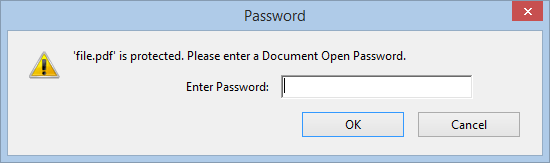In case you need to import the protected document, you can read how
here.

That’s it. You have a report generated in a pdf format that is ready for use.